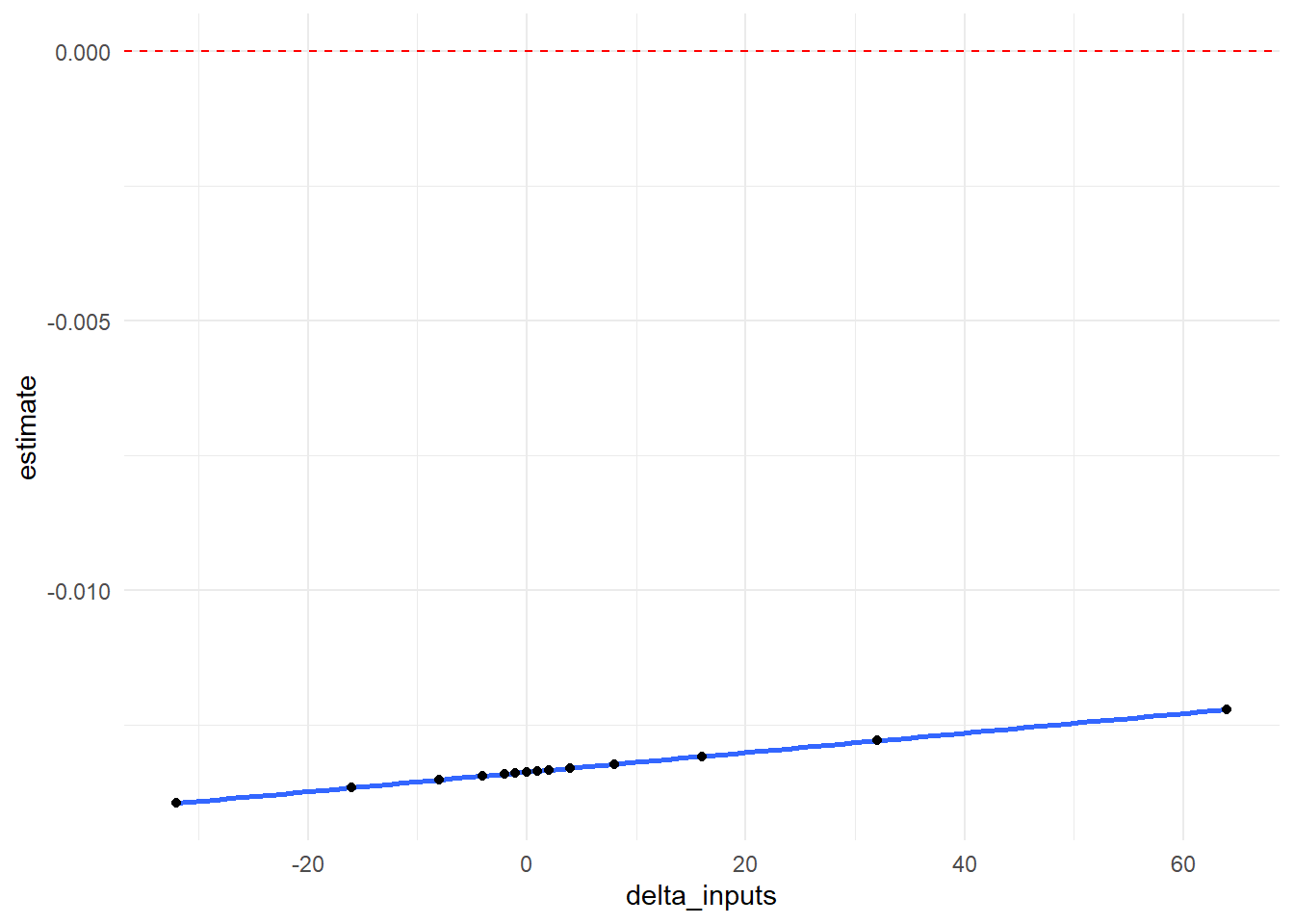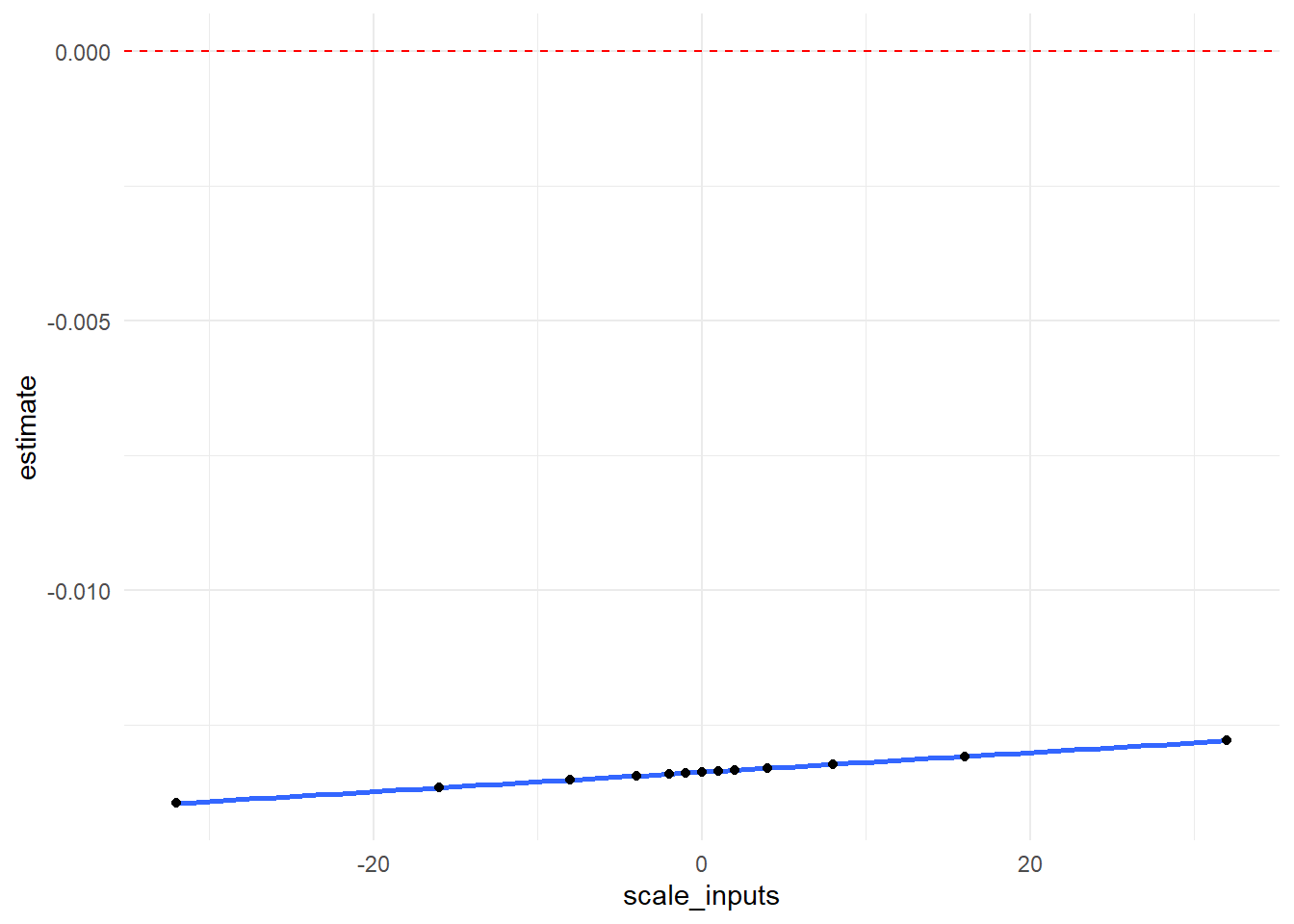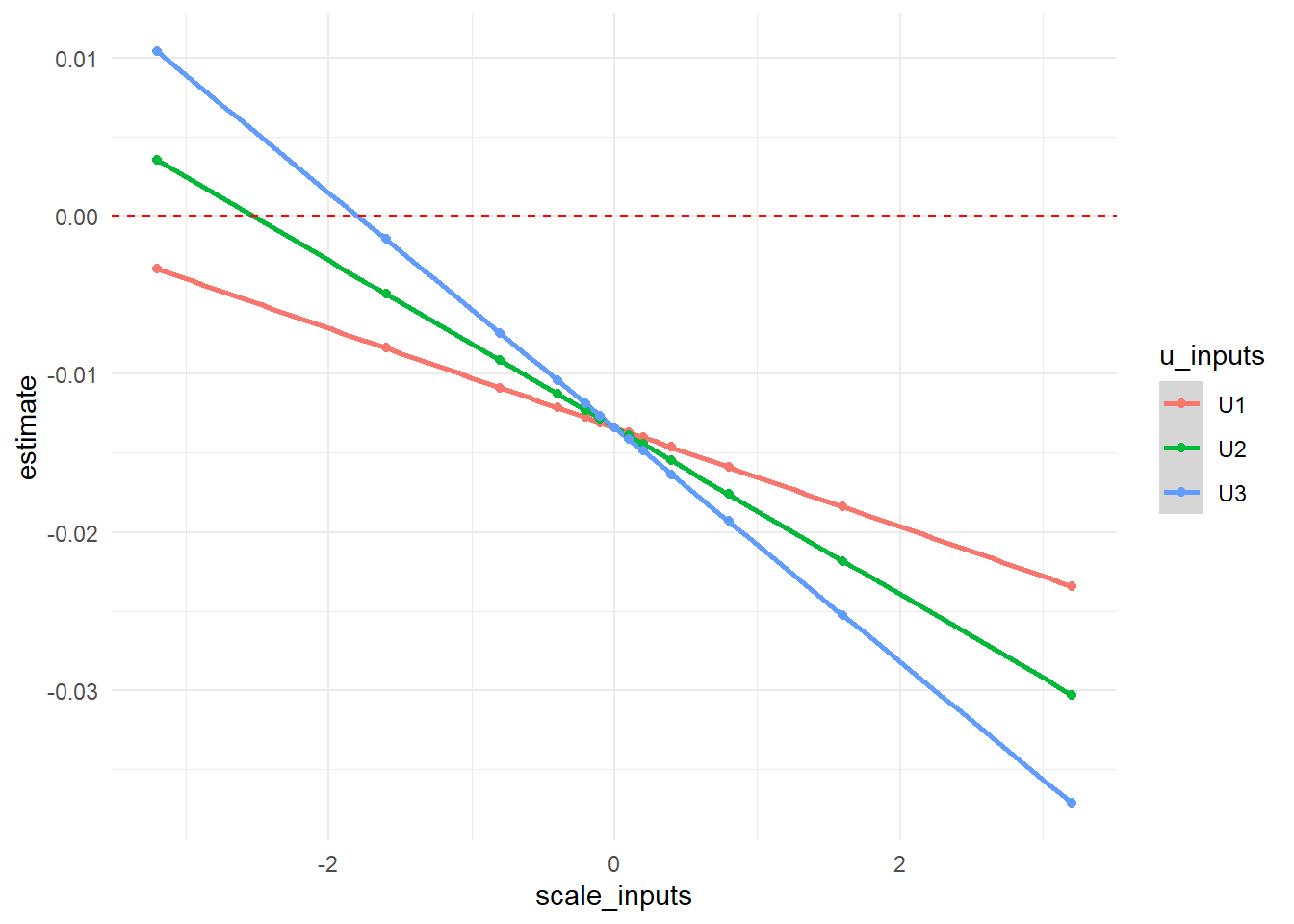# Chapter 6 Implementation in R

Note: Before beginning any sensitivity analyses, please ensure that you have created an indicator variable for loss to follow-up (i.e., outcome missingness). In this worked example, the missingness indicator is named na_flag.

## Basic descriptive statistics

First, get to know your data by assess the degree of loss to follow-up and outcome distribution among complete cases in your dataset.

• Number of observations in dataset: nrow(HRS_df) = 9253

## 6.2 Scenario 2: Loss to follow-up differential by exposure $$A$$ and/or measured confounder $$C$$ (MAR)DAG Scenario 2 (MAR)

Approach: Under an MAR missing data mechanism, we assume that study participants not lost to follow-up are exchangeable with those lost to follow-up, conditional on measured variables (exposure and/or covariates). Below, we use multiple imputation to impute our missing outcome values, using all available variables in our dataset (except our outcome missingness indicator). Other multiple imputation methods that account for the uncertainty of imputed values (e.g. joint multivariate normal imputation) may also be used.

MAR_step = mice(HRS_df, m=5, method = "norm.predict", quickpred(HRS_df, exc = "na_flag"), seed = 918, ridge = 0.0001)
# Note that variables can be included in/excluded from the imputation within the quickpred argument*
MAR_imputation =
with(MAR_step, lm(paa ~ rawwealth + age + sex + raracem))
broom::tidy(pool(MAR_imputation), conf.int = T) %>%
select(term, estimate, std.error, statistic, p.value, conf.low, conf.high) %>%
filter(term == "rawwealth") %>%
knitr::kable(bootstrap_options = "condensed")
term estimate std.error statistic p.value conf.low conf.high
rawwealth -0.0133753 0.0011294 -11.84303 0 -0.0155891 -0.0111614

#### Iterating over a range of values

Having demonstrated the logic underlying a single case, we now proceed to iterate our sensitivity analysis over a range of $$\delta$$ values. See Section 5 for a discussion on how to select a range of values to test.

To iterate over a range of $$\delta$$ values, we proceed as follows (each step is labelled in the code below):

1. We write a function with the delta parameter as the function input.

2. We create a vector specifying a range of plausible delta values

3. We run this vector of plausible delta values through the function written in Step 1.

# Write function to obtain estimate under given delta parameter
delta_MNAR_f = function(delta){

MAR_step = mice(HRS_df, m=5, method = "pmm", quickpred(HRS_df, exc = "na_flag"), seed = 918)

MNAR_conversion_delta =
complete(MAR_step, action='long', include=TRUE) %>%
mutate(paa = ifelse(na_flag == 1, paa + delta, paa))

MNAR_data_delta = as.mids(MNAR_conversion_delta)

output_delta =
with(MNAR_data_delta, lm(paa ~ rawwealth + age + sex + raracem))

params_delta =
summary(pool(output_delta)) %>%
filter(term == "rawwealth")

desc_delta = with(MNAR_data_delta, expr=c("Y_mean"=mean(paa), "Y_sd"=stats::sd(paa)))
desc_delta = withPool_MI(desc_delta) %>% as.data.frame() %>% t()

cbind(params_delta, desc_delta)

}

# Set range of plausible delta values
delta_inputs = c(-32, -16, -8, -4, -2, -1, 0, 1, 2, 4, 8, 16, 32, 64)

# Map output
output_delta =
map_df(delta_inputs, delta_MNAR_f)

rownames(output_delta) <- c()

#### Output

We can show our output in tabular and graphical form, as follows. In the output table, note that we have added two additional columns showing the mean and standard deviation of each outcome under each MNAR condition:

output_formatted_delta =
cbind(delta_inputs, output_delta) %>%
select(-term) %>%
mutate(across(c("estimate":"std.error"), round, 3),
across(c("statistic":"df", "Y_mean":"Y_sd"), round, 2),
p.value = scientific(p.value, digits = 2))

output_formatted_delta %>%
knitr::kable(bootstrap_options = "condensed")
delta_inputs estimate std.error statistic df p.value Y_mean Y_sd
-32 -0.014 0.002 -7.18 9022.21 7.5e-13 -4.45 14.41
-16 -0.014 0.001 -9.86 8483.75 0.0e+00 -1.99 10.37
-8 -0.014 0.001 -11.21 8014.03 0.0e+00 -0.76 9.08
-4 -0.013 0.001 -11.62 7830.24 0.0e+00 -0.14 8.73
-2 -0.013 0.001 -11.72 7778.00 0.0e+00 0.16 8.64
-1 -0.013 0.001 -11.73 7764.44 0.0e+00 0.32 8.62
0 -0.013 0.001 -11.73 7759.80 0.0e+00 0.47 8.61
1 -0.013 0.001 -11.70 7764.18 0.0e+00 0.62 8.62
2 -0.013 0.001 -11.66 7777.48 0.0e+00 0.78 8.64
4 -0.013 0.001 -11.50 7829.25 0.0e+00 1.08 8.73
8 -0.013 0.001 -10.97 8012.40 0.0e+00 1.70 9.08
16 -0.013 0.001 -9.45 8482.15 0.0e+00 2.93 10.36
32 -0.013 0.002 -6.59 9021.72 4.7e-11 5.39 14.39
64 -0.012 0.003 -3.65 9212.80 2.6e-04 10.31 24.63

In graphical form, we show how our $$RR$$ point estimate changes at each value of $$\delta$$. The null value of 0 is indicated with a red dotted line.

output_delta %>%
ggplot(aes(x = delta_inputs, y = estimate)) +
geom_smooth() +
geom_point() +
geom_hline(yintercept=0, linetype="dashed", color = "red") +
theme_minimal()### Scenario 3b: Multiplicative relationship between Y and data missingness ($$c$$ scale method)

#### Use case

• In this scenario, we assume that people who are lost to follow-up have 1.5 times the biological-age advancement compared to people who are not lost to follow-up

• We integrate this “prior” into our model by multiplying all imputed values of biological-age advancement in our dataset by 1.5 (using an ifelse statement conditional on our missingness indicator variable na_flag)

• Finally, we proceed to estimate our pooled effect-size parameter of interest (in this case, the risk ratio) in the same way we generated our previous multiply imputed dataset under an MAR assumption

MNAR_conversion_3b =
complete(MAR_step, action='long', include=TRUE) %>%
mutate(paa = ifelse(na_flag == 1, paa*1.5, paa))

MNAR_data_3b = as.mids(MNAR_conversion_3b)

MNAR_output_3b =
with(MNAR_data_3b, lm(paa ~ rawwealth + age + sex + raracem))

params_3b =
broom::tidy(pool(MNAR_output_3b), conf.int = T) %>%
select(term, estimate, std.error, statistic, p.value, conf.low, conf.high) %>%
filter(term == "rawwealth")

desc_3b = with(MNAR_data_3b, expr=c("Y_mean"=mean(paa), "Y_sd"=stats::sd(paa)))
desc_3b = withPool_MI(desc_3b) %>% as.data.frame() %>% t()

rownames(desc_3b) <- c()

cbind(params_3b, desc_3b) %>%
knitr::kable(bootstrap_options = "condensed") 
term estimate std.error statistic p.value conf.low conf.high Y_mean Y_sd
rawwealth -0.014446 0.0012203 -11.83781 0 -0.0168381 -0.0120539 0.5037383 9.262329

We find that $$RR=-0.0145$$. Assuming that people who are lost to follow-up have 1.5 times the biological-age advancement of to people who are not lost to follow-up, and adjusting for age, race, and sex, a $10,000 increase in total wealth is associated with a 0.015 year decrease in biological-age advancement (95%CI: -0.0169,-0.0120). #### Iterating over a range of values Having demonstrated the logic underlying a single case, we now proceed to iterate our sensitivity analysis over a range of $$c$$ values. See Section 5 for a discussion on how to select a range of values to test. To iterate over a range of $$c$$ values, we proceed as follows (each step is labelled in the code below): 1. We write a function with the scale parameter $$c$$ as the function input. 2. We create a vector specifying a range of plausible scale values $$c$$ 3. We run this vector of plausible scale values through the function written in Step 1. # Write function to obtain estimate under given scale parameter scale_MNAR_f = function(scale){ MAR_step = mice(HRS_df, m=5, method = "pmm", quickpred(HRS_df, exc = "na_flag"), seed = 918) MNAR_conversion_scale = complete(MAR_step, action='long', include=TRUE) %>% mutate(paa = ifelse(na_flag == 1, paa + scale, paa)) MNAR_data_scale = as.mids(MNAR_conversion_scale) output_scale = with(MNAR_data_scale, lm(paa ~ rawwealth + age + sex + raracem)) params_scale = summary(pool(output_scale)) %>% filter(term == "rawwealth") desc_scale = with(MNAR_data_scale, expr=c("Y_mean"=mean(paa), "Y_sd"=stats::sd(paa))) desc_scale = withPool_MI(desc_scale) %>% as.data.frame() %>% t() cbind(params_scale, desc_scale) } # Set range of plausible scale values scale_inputs = c(-32, -16, -8, -4, -2, -1, 0, 1, 2, 4, 8, 16, 32) # Map output output_scale = map_df(scale_inputs, scale_MNAR_f) rownames(output_scale) <- c() #### Output We can show our output in tabular and graphical form, as follows. In the output table, note that we have added two additional columns showing the mean and standard deviation of each outcome under each MNAR condition: output_formatted_scale = cbind(scale_inputs, output_scale) %>% select(-term) %>% mutate(across(c("estimate":"std.error"), round, 3), across(c("statistic":"df", "Y_mean":"Y_sd"), round, 2), p.value = scientific(p.value, digits = 2)) output_formatted_scale %>% knitr::kable(bootstrap_options = "condensed") scale_inputs estimate std.error statistic df p.value Y_mean Y_sd -32 -0.014 0.002 -7.18 9022.21 7.5e-13 -4.45 14.41 -16 -0.014 0.001 -9.86 8483.75 0.0e+00 -1.99 10.37 -8 -0.014 0.001 -11.21 8014.03 0.0e+00 -0.76 9.08 -4 -0.013 0.001 -11.62 7830.24 0.0e+00 -0.14 8.73 -2 -0.013 0.001 -11.72 7778.00 0.0e+00 0.16 8.64 -1 -0.013 0.001 -11.73 7764.44 0.0e+00 0.32 8.62 0 -0.013 0.001 -11.73 7759.80 0.0e+00 0.47 8.61 1 -0.013 0.001 -11.70 7764.18 0.0e+00 0.62 8.62 2 -0.013 0.001 -11.66 7777.48 0.0e+00 0.78 8.64 4 -0.013 0.001 -11.50 7829.25 0.0e+00 1.08 8.73 8 -0.013 0.001 -10.97 8012.40 0.0e+00 1.70 9.08 16 -0.013 0.001 -9.45 8482.15 0.0e+00 2.93 10.36 32 -0.013 0.002 -6.59 9021.72 4.7e-11 5.39 14.39 In graphical form, we show how our $$RR$$ point estimate changes at each value of $$\delta$$. The null value of 0 is indicated with a red dotted line. output_scale %>% ggplot(aes(x = scale_inputs, y = estimate)) + geom_smooth() + geom_point() + geom_hline(yintercept=0, linetype="dashed", color = "red") + theme_minimal()## 6.4 Scenario 4: Loss to follow-up differential on unmeasured confounder (MNAR)DAG Scenario 4 (MNAR) Under an MNAR missing data mechanism where we loss to follow-up is conditional on the outcome, we assume that study participants lost to follow-up are not exchangeable with those not lost to follow-up; the true relationship between exposure and outcome differs between the two groups based on an unmeasured common cause of exposure and disease. In thie case, there are the following relationships to specify: • The confounder-exposure relationship • The confounder-disease relationship • The confounder-missingness relationship Below, we show the implementation of sensitivity analyses for an unmeasured confounder causing Y as follows: 1. We generate several unmeasured confounders using the MAR-imputed dataset (this serves to vary the $$A-U$$ and $$Y-U$$ relationship) 2. We create a vector of offset terms to define the relationship between U and missingness (this serves to vary the $$U-LTFU$$ relationship) 3. We provide example code to iterate over these unmeasured confounders to demonstrate their effect on the causal effect estimate given a range of associations between each confounder and outcome missingness ### Example and sample code #### Generating unmeasured confounders: We generate an unmeasured confounder $$U$$, expressed as a function of both exposure $$A$$ and disease $$Y$$ (Generating the confounder variable as a function of exposure and disease might seem strange, as it would seem that we are creating a collider variable. However, because we are hypothesizing in our sensitivity analyses that the observed $$A-Y$$ relationship is not the “true” causal effect, we can generate U without concerning ourselves with directionality; the magnitude of the $$A-U$$ and $$Y-U$$ relationships are our primary concern.) Below, we create several potential unmeasured confounders ($$U1$$, $$U2$$, $$U3$$) as a function of exposure and disease in our MAR-imputed dataset. You may create as many $$U$$ variables as desired. MAR_step = mice(HRS_df, m=5, method = "pmm", quickpred(HRS_df, exc = "na_flag"), seed = 918) imputed_long_df = complete(MAR_step, action='long', include=TRUE) %>% mutate(U1 = (((rawwealth+15000)/5000) + (1.5*paa)), U2 = (((rawwealth+15000)/5000) + (2.5*paa)), U3 = (((rawwealth+15000)/5000) + (3.5*paa)) ) After having generated the variable, we generate descriptive statistics for $$U$$ and test $$U$$-exposure and $$U$$-disease relationships: imputed_MAR_df = as.mids(imputed_long_df) # Calculate mean of outcome and unmeasured confounders using imputed data desc_U = with(imputed_MAR_df, expr=c("Y_mean"=mean(paa), "Y_sd"=sd(paa), "U1_mean"=mean(U1), "U1_sd"=sd(U1), "U2_mean"=mean(U2), "U2_sd"=sd(U2), "U3_mean"=mean(U3), "U3_sd"=sd(U3))) desc_U = withPool_MI(desc_U) %>% as.data.frame() desc_U %>% knitr::kable(caption = "Mean and standard deviation of outcome and unmeasured confounders") Table 6.1: Mean and standard deviation of outcome and unmeasured confounders . Y_mean 0.4700495 Y_sd 8.6107036 U1_mean 3.7127219 U1_sd 12.9141038 U2_mean 4.1827714 U2_sd 21.5248036 U3_mean 4.6528209 U3_sd 30.1355056 # Calculate exposure-confounder associations for all U variables EC_model_U1 = with(imputed_MAR_df, lm(rawwealth ~ U1)) EC_model_U1 = summary(pool(EC_model_U1)) %>% filter(term == "U1") EC_model_U2 = with(imputed_MAR_df, lm(rawwealth ~ U2)) EC_model_U2 = summary(pool(EC_model_U2)) %>% filter(term == "U2") EC_model_U3 = with(imputed_MAR_df, lm(rawwealth ~ U3)) EC_model_U3 = summary(pool(EC_model_U3)) %>% filter(term == "U3") EC_models_summary = rbind(EC_model_U1, EC_model_U2) EC_models_summary = rbind(EC_models_summary, EC_model_U3) EC_models_summary %>% select(term, estimate, std.error) %>% rename(variable = term, RR = estimate) %>% knitr::kable(bootstrap_options = "condensed", caption = "Risk Ratios, U-A relationship") Table 6.1: Risk Ratios, U-A relationship variable RR std.error U1 -0.7520250 0.0625914 U2 -0.4529040 0.0375505 U3 -0.3240197 0.0268205 # Calculate disease-confounder associations for all U variables DC_model_U1 = with(imputed_MAR_df, lm(paa ~ U1)) DC_model_U1 = summary(pool(DC_model_U1)) %>% filter(term == "U1") DC_model_U2 = with(imputed_MAR_df, lm(paa ~ U2)) DC_model_U2 = summary(pool(DC_model_U2)) %>% filter(term == "U2") DC_model_U3 = with(imputed_MAR_df, lm(paa ~ U3)) DC_model_U3 = summary(pool(DC_model_U3)) %>% filter(term == "U3") DC_models_summary = rbind(DC_model_U1, DC_model_U2) DC_models_summary = rbind(DC_models_summary, DC_model_U3) DC_models_summary %>% select(term, estimate, std.error) %>% rename(variable = term, RR = estimate) %>% knitr::kable(bootstrap_options = "condensed", caption = "Risk Ratios, U-Y relationship") Table 6.1: Risk Ratios, U-Y relationship variable RR std.error U1 0.6667669 8.3e-06 U2 0.4000362 3.0e-06 U3 0.2857328 1.5e-06 #### Defining the confounder-missingness relationship As in scenario 6.3, we then modify imputed outcome values by an offset parameter (which is represented as a function of our unmeasured confounder): # Write function to obtain estimate under given scale parameter MNAR_U_f = function(scale, u_var){ imputed_long_df = imputed_long_df %>% mutate(paa = ifelse(na_flag == 1, paa + scale*pull(imputed_long_df, u_var), paa)) MNAR_data_scale = as.mids(imputed_long_df) output_scale = with(MNAR_data_scale, lm(paa ~ rawwealth + age + sex + raracem)) params_scale = summary(pool(output_scale)) %>% filter(term == "rawwealth") desc_scale = with(MNAR_data_scale, expr=c("Y_mean"=mean(paa), "Y_sd"=stats::sd(paa))) desc_scale = withPool_MI(desc_scale) %>% as.data.frame() %>% t() cbind(params_scale, desc_scale) } # Set range of plausible scale values scale_inputs = c(-3.2, -1.6, -.8, -.4, -.2, -.1, 0, .1, .2, .4, .8, 1.6, 3.2) # Create vector of all U variables u_inputs = c("U1", "U2", "U3") # Create cross of those two vectors for model inputs MNAR_U_inputs = crossing(u_inputs, scale_inputs) # Map and format output output_U = map2_df(MNAR_U_inputs$scale_inputs, MNAR_U_inputs\$u_inputs, MNAR_U_f)

output_U =
cbind(MNAR_U_inputs, output_U)

### Output

We can show our output in tabular and graphical form, as follows. Note that we have added two additional columns showing the mean and standard deviation of each outcome under each MNAR condition:

output_U_formatted =
output_U %>%
select(-term) %>%
mutate(across(c("estimate":"std.error"), round, 3),
across(c("statistic":"df", "Y_mean", "Y_sd"), round, 2),
p.value = scientific(p.value, digits = 2))

rownames(output_U_formatted) <- c()

output_U_formatted %>%
knitr::kable(bootstrap_options = "condensed")
u_inputs scale_inputs estimate std.error statistic df p.value Y_mean Y_sd
U1 -3.2 -0.003 0.002 -1.57 2225.66 1.2e-01 -1.34 15.35
U1 -1.6 -0.008 0.001 -6.51 6370.78 7.9e-11 -0.43 9.43
U1 -0.8 -0.011 0.001 -10.11 9237.88 0.0e+00 0.02 8.06
U1 -0.4 -0.012 0.001 -11.31 9175.19 0.0e+00 0.24 8.09
U1 -0.2 -0.013 0.001 -11.62 8756.31 0.0e+00 0.36 8.29
U1 -0.1 -0.013 0.001 -11.70 8326.93 0.0e+00 0.41 8.44
U1 0.0 -0.013 0.001 -11.73 7759.80 0.0e+00 0.47 8.61
U1 0.1 -0.014 0.001 -11.72 7102.98 0.0e+00 0.53 8.81
U1 0.2 -0.014 0.001 -11.68 6416.81 0.0e+00 0.58 9.03
U1 0.4 -0.015 0.001 -11.52 5146.95 0.0e+00 0.70 9.54
U1 0.8 -0.016 0.001 -11.00 3435.11 0.0e+00 0.92 10.78
U1 1.6 -0.018 0.002 -9.82 2104.01 0.0e+00 1.37 13.83
U1 3.2 -0.023 0.003 -8.14 1500.01 8.9e-16 2.28 20.99
U2 -3.2 0.004 0.003 1.02 1465.29 3.1e-01 -1.56 24.68
U2 -1.6 -0.005 0.002 -2.77 2622.85 5.7e-03 -0.54 12.86
U2 -0.8 -0.009 0.001 -7.79 7898.30 7.5e-15 -0.04 8.69
U2 -0.4 -0.011 0.001 -10.61 9243.86 0.0e+00 0.22 7.99
U2 -0.2 -0.012 0.001 -11.44 9092.04 0.0e+00 0.34 8.14
U2 -0.1 -0.013 0.001 -11.65 8629.81 0.0e+00 0.41 8.34
U2 0.0 -0.013 0.001 -11.73 7759.80 0.0e+00 0.47 8.61
U2 0.1 -0.014 0.001 -11.71 6643.99 0.0e+00 0.53 8.95
U2 0.2 -0.014 0.001 -11.60 5538.38 0.0e+00 0.60 9.36
U2 0.4 -0.015 0.001 -11.23 3867.76 0.0e+00 0.72 10.32
U2 0.8 -0.018 0.002 -10.28 2341.25 0.0e+00 0.98 12.69
U2 1.6 -0.022 0.003 -8.72 1551.69 0.0e+00 1.48 18.33
U2 3.2 -0.030 0.004 -7.12 1274.12 1.8e-12 2.50 30.83
U3 -3.2 0.010 0.005 2.15 1296.35 3.2e-02 -1.78 34.67
U3 -1.6 -0.001 0.002 -0.62 1770.58 5.3e-01 -0.65 17.22
U3 -0.8 -0.007 0.001 -5.44 4750.70 5.7e-08 -0.09 9.99
U3 -0.4 -0.010 0.001 -9.61 9177.70 0.0e+00 0.19 8.10
U3 -0.2 -0.012 0.001 -11.17 9218.57 0.0e+00 0.33 8.03
U3 -0.1 -0.013 0.001 -11.58 8864.18 0.0e+00 0.40 8.25
U3 0.0 -0.013 0.001 -11.73 7759.80 0.0e+00 0.47 8.61
U3 0.1 -0.014 0.001 -11.68 6187.66 0.0e+00 0.54 9.11
U3 0.2 -0.015 0.001 -11.48 4768.74 0.0e+00 0.61 9.72
U3 0.4 -0.016 0.002 -10.88 3040.69 0.0e+00 0.75 11.20
U3 0.8 -0.019 0.002 -9.61 1854.99 0.0e+00 1.03 14.81
U3 1.6 -0.025 0.003 -7.95 1359.10 4.0e-15 1.59 23.14
U3 3.2 -0.037 0.006 -6.55 1203.58 8.3e-11 2.72 40.99

In graphical form, we show how our $$RR$$ point estimate changes at each value of $$\delta$$ with each potential unmeasured confounder as a different line. The null value of 0 is indicated with a red dotted line.

output_U %>%
ggplot(aes(x = scale_inputs, y = estimate, color = u_inputs)) +
geom_smooth() +
geom_point() +
geom_hline(yintercept=0, linetype="dashed", color = "red") +
theme_minimal()